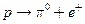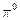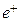Chapter 33, Problem 37PE

Chapter
Section
Textbook Problem

(a) How much energy would be released if the proton did decay 1uria the conjectured reaction(b) Given that thedecays to two (s and that thewill find an electron to annihilate, what total energy is ultimately produced in proton decay?(c) Why is this energy greater than the proton's total mass (converted to energy)?

To determine

(a)

The energy released in the decay of proton given by pπ0+e+

Explanation

Given info:

The decay of proton is given by, pπ0+e+

Formula used:

Energy released = Rest energy of initial particle − sum of energies of decay products

Calculation:

In this problem the proton decays by pπ0+e+

Rest energy of proton =938 MeV

Rest en

To determine

(b)

The ultimate release of energy if the decay product π0 of the reaction pπ0+e+ is again

decayed by π0γ+γ and positron is annihilated with an electron.

To determine

(c)

Why total amount of energy released is greater than proton's total mass.

Still sussing out bartleby?

Check out a sample textbook solution.

See a sample solution

The Solution to Your Study Problems

Bartleby provides explanations to thousands of textbook problems written by our experts, many with advanced degrees!

Get Started